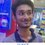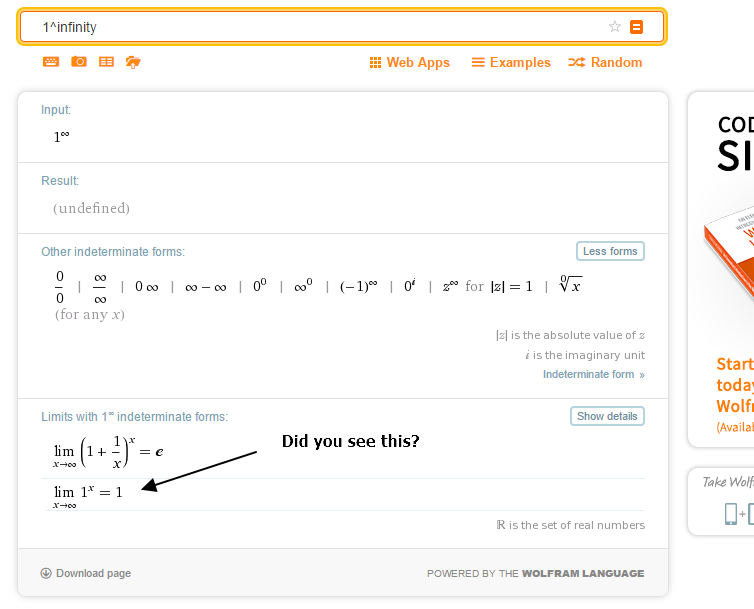# Unity is confusing!

$\huge 1^{1+2+3+4+5+6+7\cdots}$

Considering the fact that some diverging sums can also approach a certain limit. What is the sum of real value(s) of the expression above?

Details:

• If no real value(s) are obtained give your answer as $Not$ $defined$.

• If the limit approaches $\infty$ then enter your answer as $\infty$ as well.

This problem is original. Upon pondering over the answer to this problem I wasn't able to come up with a legit explanation as to why my method was/wasn't correct. Please enter your answer with an appropriate explanation.Note by Tapas Mazumdar
3 years, 5 months ago

This discussion board is a place to discuss our Daily Challenges and the math and science related to those challenges. Explanations are more than just a solution — they should explain the steps and thinking strategies that you used to obtain the solution. Comments should further the discussion of math and science.

When posting on Brilliant:

• Use the emojis to react to an explanation, whether you're congratulating a job well done , or just really confused .
• Ask specific questions about the challenge or the steps in somebody's explanation. Well-posed questions can add a lot to the discussion, but posting "I don't understand!" doesn't help anyone.
• Try to contribute something new to the discussion, whether it is an extension, generalization or other idea related to the challenge.

MarkdownAppears as
*italics* or _italics_ italics
**bold** or __bold__ bold
- bulleted- list
• bulleted
• list
1. numbered2. list
1. numbered
2. list
Note: you must add a full line of space before and after lists for them to show up correctly
paragraph 1paragraph 2

paragraph 1

paragraph 2

[example link](https://brilliant.org)example link
> This is a quote
This is a quote
    # I indented these lines
# 4 spaces, and now they show
# up as a code block.

print "hello world"
# I indented these lines
# 4 spaces, and now they show
# up as a code block.

print "hello world"
MathAppears as
Remember to wrap math in $$ ... $$ or $ ... $ to ensure proper formatting.
2 \times 3 $2 \times 3$
2^{34} $2^{34}$
a_{i-1} $a_{i-1}$
\frac{2}{3} $\frac{2}{3}$
\sqrt{2} $\sqrt{2}$
\sum_{i=1}^3 $\sum_{i=1}^3$
\sin \theta $\sin \theta$
\boxed{123} $\boxed{123}$

Sort by:

all i see is an infinite number of 1's being multiplied.

- 3 years, 5 months ago

We are essentially calculating $\displaystyle \lim_{n\to\infty} 1^{n(n+1)/2} = 1^\infty = 1$.

- 3 years, 5 months ago

Even though the limit equals 1, the equality $1^\infty=1$ you mention is incorrect since $1^\infty$ is indeterminate.

- 3 years, 4 months ago

It's correct. The link you gave me have the limit in the form of $(f(x))^{g(x)}$, where $f(x)$ is a non-constant function, whereas my $f(x)$ is a constant function of 1.

- 3 years, 4 months ago

Well then, here's a different link to support my statement. Also, this.

- 3 years, 4 months ago- 3 years, 4 months ago

I did. I never said that the limit doesn't equals 1. In fact, my first reply to you on this thread states "Even though the limit equals 1, the equality..."

I'm disputing the parts $1^\infty=1$ and $\lim\limits_{n\to\infty}1^{n(n+1)/2}=1^\infty$ in your comment. You should write it more like $\lim\limits_{n\to\infty}1^{n(n+1)/2}=\lim\limits_{n\to\infty}1=1$ where the second equality follows from the fact that $1^x=1$ for any real $x$.

- 3 years, 4 months ago

Ah sorry, I didnt' see what I wrote. It's been a long time. Good eye!

Is it just me, but I tried editing my comment but I can't seem to do it. Weird....

- 3 years, 4 months ago

Yes, that's a known bug. Fortunately, there's a way around it if you're on PC. I posted the method in #bug-report of Brilliant Lounge a few days ago. You can check it out if you want.

- 3 years, 4 months ago

Also for any real number $n$ (say) we can say that:

$\displaystyle \lim_{x\to\infty}{\large\sqrt[x]{n}} = 1$

$\because$ The above can be written as $\displaystyle \lim_{x\to\infty}{\large n^{\frac{1}{x}}}$.

$\therefore$ As $x\to\infty$, $\dfrac{1}{x}\to 0$, hence limit of the function reaches $1$.

So, if $\sqrt[\infty]{n}=1$ (as in most cases, the limit and the value mean quite the same thing), can't we say that $1^{\infty} = n$, which is kind of a paradox. And the only plausible explanation to this is that $1^{\infty}$ is Not defined.

But if we follow $\zeta\left(-1\right)=\dfrac{-1}{12}$, then this turns out to be $\large1^{\frac{-1}{12}}$ which I'm confused about because the $12$th root of $1$ is both $1$ and $-1$ (or is it just 1?) and $10$ complex roots.

So, what can be the answer?

- 3 years, 5 months ago

Read up indetermediate forms, you did not obey those rules, so your logic is incorrect.

Plus, if you want to invoke 1+2+ 3 + ... = -1/12, then you should make it clear that you're using riemann zeta regularization from the start. Otherwise, by convention, 1 +2 + 3 + ... = infinity

- 3 years, 5 months ago

But the string theory tells us that $\displaystyle \sum_{n=1}^{\infty}{n}~ \left(\text{or}\right)~ \zeta\left(-1\right) = \dfrac{-1}{12}$. Wouldn't that concept be counted right here?

- 3 years, 5 months ago

Think about how zeta(-1) = -1/12 was derived in the first place. Did it apply Abel sum? Read up sums of divergent series.

- 3 years, 5 months ago